# DL-SCH and PDSCH Transmit and Receive Processing Chain

This example shows how to use 5G Toolbox™ features to model a 5G NR physical downlink shared channel (PDSCH) link, including all of the steps from transport block generation to bit decoding at the receiver end.

### Introduction

This diagram shows the downlink shared channel (DL-SCH) and PDSCH transmit and receive processing chain.This example shows how to model these elements of a link-level simulation.

• DL-SCH encoding

• Hybrid ARQ (HARQ) management

• PDSCH encoding

• Multiple-input multiple-output (MIMO) precoding

• OFDM modulation

• Propagation channel and noise addition

• Timing synchronization

• OFDM demodulation

• Channel estimation and equalization

• PDSCH decoding

• DL-SCH decoding

For an example of how to use link-level simulation to measure throughput, see NR PDSCH Throughput.

### Simulation Parameters

Specify the signal-to-noise ratio (SNR), number of slots to simulate, and perfect channel estimation flag. To learn more about the SNR definition used in this example, see SNR Definition Used in Link Simulations.

```SNRdB = 10; % SNR in dB totalNoSlots = 20; % Number of slots to simulate perfectEstimation = false; % Perfect synchronization and channel estimation rng("default"); % Set default random number generator for repeatability```

### Carrier Configuration

Create a carrier configuration object. This object controls the numerology, such as, the subcarrier spacing, bandwidth, and cyclic prefix (CP) length. This example uses the default set of properties.

`carrier = nrCarrierConfig`
```carrier = nrCarrierConfig with properties: NCellID: 1 SubcarrierSpacing: 15 CyclicPrefix: 'normal' NSizeGrid: 52 NStartGrid: 0 NSlot: 0 NFrame: 0 Read-only properties: SymbolsPerSlot: 14 SlotsPerSubframe: 1 SlotsPerFrame: 10 ```

### PDSCH and DM-RS Configuration

Create a PDSCH configuration object. Specify the modulation scheme (16-QAM) and the number of layers (2). Allocate all resource blocks (RBs) to the PDSCH (full band allocation). You can also specify other time-allocation parameters and demodulation reference signal (DM-RS) settings in this object.

```pdsch = nrPDSCHConfig; pdsch.Modulation = "16QAM"; pdsch.NumLayers = 2; pdsch.PRBSet = 0:carrier.NSizeGrid-1; % Full band allocation```

Display the PDSCH parameters.

`pdsch`
```pdsch = nrPDSCHConfig with properties: NSizeBWP: [] NStartBWP: [] ReservedPRB: {[1x1 nrPDSCHReservedConfig]} ReservedRE: [] Modulation: '16QAM' NumLayers: 2 MappingType: 'A' SymbolAllocation: [0 14] PRBSet: [0 1 2 3 4 5 6 7 8 9 10 11 12 13 14 15 16 17 ... ] VRBToPRBInterleaving: 0 VRBBundleSize: 2 NID: [] RNTI: 1 DMRS: [1x1 nrPDSCHDMRSConfig] EnablePTRS: 0 PTRS: [1x1 nrPDSCHPTRSConfig] Read-only properties: NumCodewords: 1 ```

Set the DM-RS parameters. To improve channel estimation, add an additional DM-RS position.

`pdsch.DMRS.DMRSAdditionalPosition = 1;`

Set the DM-RS configuration type and the DM-RS length, which determines the number of orthogonal DM-RS sequences or DM-RS ports.

• `DMRSConfigurationType = 1 `supports up to 4 DM-RS ports when `DMRSLength = 1`.

• `DMRSConfigurationType = 1 `supports up to 8 DM-RS ports when `DMRSLength = 2`.

• `DMRSConfigurationType = 2 `supports up to 6 DM-RS ports when `DMRSLength = 1`. This is designed for multi-user MIMO (MU-MIMO).

• `DMRSConfigurationType = 2 `supports up to 12 DM-RS ports when `DMRSLength = 2`. This is designed for MU-MIMO.

The maximum number of layers must be less than or equal to the number of DM-RS ports.

```pdsch.DMRS.DMRSConfigurationType = 1; pdsch.DMRS.DMRSLength = 2; pdsch.DMRS % Display DM-RS properties```
```ans = nrPDSCHDMRSConfig with properties: DMRSConfigurationType: 1 DMRSReferencePoint: 'CRB0' DMRSTypeAPosition: 2 DMRSAdditionalPosition: 1 DMRSLength: 2 CustomSymbolSet: [] DMRSPortSet: [] NIDNSCID: [] NSCID: 0 NumCDMGroupsWithoutData: 2 DMRSDownlinkR16: 0 Read-only properties: CDMGroups: [0 0] DeltaShifts: [0 0] FrequencyWeights: [2x2 double] TimeWeights: [2x2 double] DMRSSubcarrierLocations: [6x2 double] CDMLengths: [2 1] ```

### DL-SCH Configuration

Specify the code rate, the number of HARQ processes, and the redundancy version (RV) sequence values. This sequence controls the RV retransmissions in case of error. To disable HARQ retransmissions, you can set `rvSeq` to a fixed value (for example, 0). For more information on how to model transport channels with HARQ, see Model 5G NR Transport Channels with HARQ.

```NHARQProcesses = 16; % Number of parallel HARQ processes rvSeq = [0 2 3 1];```

Take into account the number of codewords when specifying the coding rate. The number of codewords is a read-only property of the PDSCH configuration object that depends on the number of layers.

• 1 codeword for up to 4 layers

• 2 codewords for more than 4 layers

```% Coding rate if pdsch.NumCodewords == 1 codeRate = 490/1024; else codeRate = [490 490]./1024; end```

Create the DL-SCH encoder and decoder objects. To use multiple processes, set the `MultipleHARQProcesses` property to `true` for both objects. You do not need to specify the number of HARQ processes. The DL-SCH encoder and decoder objects can model up to 16 HARQ processes. To identify the active HARQ process when performing operations with the DL-SCH encoder and decoder objects, use the `HARQprocessID` property of the HARQ entity object, defined in the next section.

```% Create DL-SCH encoder object encodeDLSCH = nrDLSCH; encodeDLSCH.MultipleHARQProcesses = true; encodeDLSCH.TargetCodeRate = codeRate; % Create DLSCH decoder object decodeDLSCH = nrDLSCHDecoder; decodeDLSCH.MultipleHARQProcesses = true; decodeDLSCH.TargetCodeRate = codeRate; decodeDLSCH.LDPCDecodingAlgorithm = "Normalized min-sum"; decodeDLSCH.MaximumLDPCIterationCount = 6;```

### HARQ Management

Create a HARQ entity object to manage the HARQ processes and the DL-SCH encoder and decoder buffers. For each HARQ process, a HARQ entity stores these elements:

• HARQ ID number.

• RV.

• Transmission number, which indicates how many times a certain transport block has been transmitted.

• Flag to indicate whether new data is required. New data is required when a transport block is received successfully or if a sequence timeout has occurred (all RV transmissions have failed).

• Flag to indicate whether a sequence timeout has occurred (all RV transmissions have failed).

`harqEntity = HARQEntity(0:NHARQProcesses-1,rvSeq,pdsch.NumCodewords);`

### Channel Configuration

Specify the number of transmit and receive antennas.

```nTxAnts = 8; nRxAnts = 8; % Check that the number of layers is valid for the number of antennas if pdsch.NumLayers > min(nTxAnts,nRxAnts) error("The number of layers ("+string(pdsch.NumLayers)+") must be smaller than min(nTxAnts,nRxAnts) ("+string(min(nTxAnts,nRxAnts))+")") end```

Create a channel object.

```channel = nrTDLChannel; channel.DelayProfile = "TDL-C"; channel.NumTransmitAntennas = nTxAnts; channel.NumReceiveAntennas = nRxAnts;```

Set the channel sample rate to that of the OFDM signal. To obtain the sampling rate of the OFDM signal, use the `nrOFDMInfo` function.

```ofdmInfo = nrOFDMInfo(carrier); channel.SampleRate = ofdmInfo.SampleRate;```

### Transmission and Reception

Set up a loop to simulate the transmission and reception of slots. Create a `comm.ConstellationDiagram` to display the constellation of the equalized signal.

```constPlot = comm.ConstellationDiagram; % Constellation diagram object constPlot.ReferenceConstellation = getConstellationRefPoints(pdsch.Modulation); % Reference constellation values constPlot.EnableMeasurements = 1; % Enable EVM measurements % Initial timing offset offset = 0; estChannelGrid = getInitialChannelEstimate(channel,carrier); newPrecodingWeight = getPrecodingMatrix(pdsch.PRBSet,pdsch.NumLayers,estChannelGrid); for nSlot = 0:totalNoSlots-1 % New slot carrier.NSlot = nSlot;```

#### Calculate Transport Block Size

The transport block size is the number of bits to send to the channel coding stages. This value depends on the capacity of the PDSCH. To calculate the transport block size, use the `nrTBS` function.

``` % Generate PDSCH indices info, which is needed to calculate the transport % block size [pdschIndices,pdschInfo] = nrPDSCHIndices(carrier,pdsch); % Calculate transport block sizes Xoh_PDSCH = 0; trBlkSizes = nrTBS(pdsch.Modulation,pdsch.NumLayers,numel(pdsch.PRBSet),pdschInfo.NREPerPRB,codeRate,Xoh_PDSCH);```

#### HARQ Processing (Buffer Management)

This section explains the buffer management in the encoder and decoder.

• DL-SCH encoder buffers: Generate a new transport block if new data is required for the active HARQ process. Store the transport block in the corresponding buffer. If new data is not required, the DL-SCH encoder uses its buffered bits for retransmission.

• DL-SCH decoder buffers: The soft buffers in the receiver store previously received versions of the same codeword. These buffers are cleared automatically upon successful reception (no CRC error). However, if the RV sequence ends without successful decoding, flush the buffers manually by using the `resetSoftBuffer` object function.

``` % Get new transport blocks and flush decoder soft buffer, as required for cwIdx = 1:pdsch.NumCodewords if harqEntity.NewData(cwIdx) % Create and store a new transport block for transmission trBlk = randi([0 1],trBlkSizes(cwIdx),1); setTransportBlock(encodeDLSCH,trBlk,cwIdx-1,harqEntity.HARQProcessID); % If the previous RV sequence ends without successful % decoding, flush the soft buffer if harqEntity.SequenceTimeout(cwIdx) resetSoftBuffer(decodeDLSCH,cwIdx-1,harqEntity.HARQProcessID); end end end```

#### DL-SCH Encoding

Encode the transport blocks. The transport block is already stored in one of the internal soft buffers of the DL-SCH encoder object.

` codedTrBlock = encodeDLSCH(pdsch.Modulation,pdsch.NumLayers,pdschInfo.G,harqEntity.RedundancyVersion,harqEntity.HARQProcessID);`

#### PDSCH Modulation and MIMO Precoding

Generate PDSCH symbols from the coded transport blocks.

` pdschSymbols = nrPDSCH(carrier,pdsch,codedTrBlock);`

Get the precoding weights. This example assumes channel knowledge for precoding. (For an example of how to use the channel estimate at the receiver to calculate the weights used for the transmission in the next slot, see NR PDSCH Throughput.)

` precodingWeights = newPrecodingWeight;`

Precode the PDSCH symbols.

` pdschSymbolsPrecoded = pdschSymbols*precodingWeights;`

#### PDSCH DM-RS Generation

Generate DM-RS symbols and indices.

``` dmrsSymbols = nrPDSCHDMRS(carrier,pdsch); dmrsIndices = nrPDSCHDMRSIndices(carrier,pdsch);```

#### Mapping to Resource Grid

Generate an empty resource grid. This grid represents a slot.

` pdschGrid = nrResourceGrid(carrier,nTxAnts);`

The `nrPDSCHIndices` function generates indices that refer to layers and not antennas. This format is useful when mapping PDSCH symbols directly to layers. In this case, the resulting resource grids are not precoded. This figure shows the mapping process of the PDSCH symbols to as many resource grids as layers.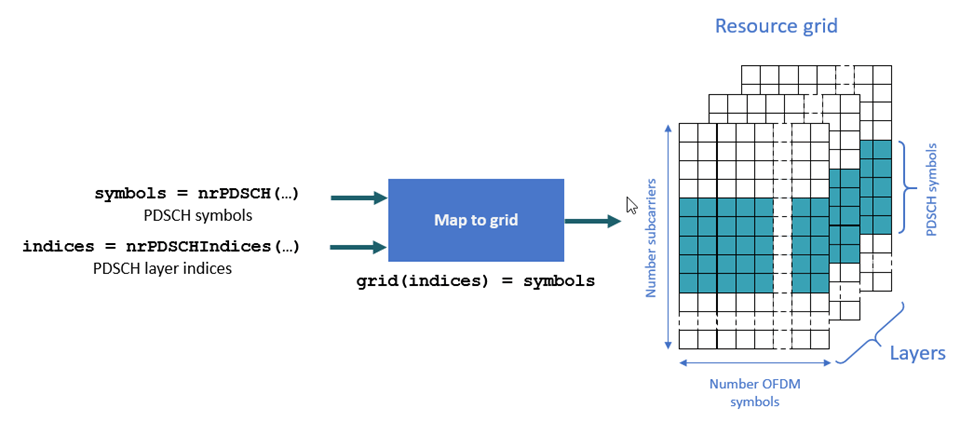Because this example applies MIMO precoding to the PDSCH symbols before mapping them to the resource grids, the MIMO-precoded PDSCH symbols refer to antennas and not layers. To convert layer indices to antenna indices, use the `nrExtractResources` function. This figure shows the mapping process of MIMO-precoded symbols to as many resource grids as transmit antennas.``` [~,pdschAntIndices] = nrExtractResources(pdschIndices,pdschGrid); pdschGrid(pdschAntIndices) = pdschSymbolsPrecoded;```

MIMO-precode and map the DM-RS symbols to the resource grid. Similar to the PDSCH indices, the DM-RS indices refer to layers. To convert these layer indices to antenna indices, use the `nrExtractResources` function again.

``` % PDSCH DM-RS precoding and mapping for p = 1:size(dmrsSymbols,2) [~,dmrsAntIndices] = nrExtractResources(dmrsIndices(:,p),pdschGrid); pdschGrid(dmrsAntIndices) = pdschGrid(dmrsAntIndices) + dmrsSymbols(:,p)*precodingWeights(p,:); end```

#### OFDM Modulation

OFDM-modulate the resource grid.

` [txWaveform,waveformInfo] = nrOFDMModulate(carrier,pdschGrid);`

#### Propagation Channel

The propagation channel generates N output samples for an input with N samples. However, the block of N output samples includes the channel filter transient (K samples). Because the synchronization stage removes this initial transient, if a slot at the channel output has N samples, N-K samples remain after synchronization. N-K samples are not enough to decode a slot-worth of data. Part of the slot samples are in the channel filter delay line and are not flushed yet. To flush all relevant samples out of the channel filter, pad the input signal with zeros. The maximum delay that the channel filter introduces affects the size of the padding. The padding accounts for the delay introduced by all multipath components and the channel filter implementation delay. This figure shows the need for zero padding before a waveform enters the channel.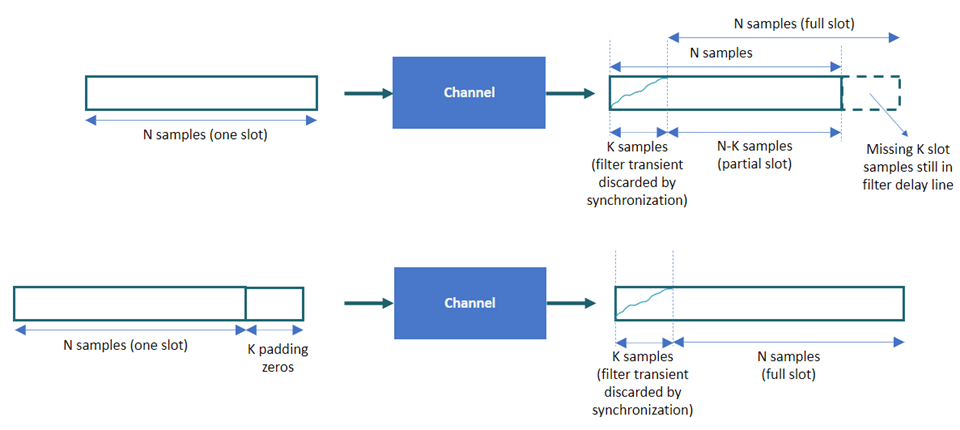Pad the input signal with enough zeros to ensure that the generated signal is flushed out of the channel filter.

``` chInfo = info(channel); maxChDelay = ceil(max(chInfo.PathDelays*channel.SampleRate)) + chInfo.ChannelFilterDelay; txWaveform = [txWaveform; zeros(maxChDelay,size(txWaveform,2))];```

Send the signal through the channel and add noise.

``` [rxWaveform,pathGains,sampleTimes] = channel(txWaveform); noise = generateAWGN(SNRdB,nRxAnts,waveformInfo.Nfft,size(rxWaveform)); rxWaveform = rxWaveform + noise;```

#### Timing Synchronization

You can perform perfect or practical synchronization.

• Perfect synchronization assumes channel knowledge (`nrPerfectTimingEstimate`). The channel returns information on path gains and path filters impulse response. You can use this information to determine the offset that is associated with the strongest multipath component across all channel snapshots and across all transmit and receive antennas.

• Practical synchronization performs a cross-correlation of the received signal with the PDSCH DM-RS symbols in the time domain (`nrTimingEstimate`). In some adverse cases, this cross-correlation can be weak due to fading or noise, resulting in an erroneous timing offset. The function `hSkipWeakTimingOffset` checks the magnitude of the cross-correlation `mag`. If the cross-correlation is weak, the function ignores the current timing estimate and instead uses the previous estimate (`offset`).

Perform perfect or practical timing estimation and synchronization.

``` if perfectEstimation % Get path filters for perfect timing estimation pathFilters = getPathFilters(channel); [offset,mag] = nrPerfectTimingEstimate(pathGains,pathFilters); else [t,mag] = nrTimingEstimate(carrier,rxWaveform,dmrsIndices,dmrsSymbols); offset = hSkipWeakTimingOffset(offset,t,mag); end rxWaveform = rxWaveform(1+offset:end,:);```

#### OFDM Demodulation

OFDM-demodulate the synchronized signal.

` rxGrid = nrOFDMDemodulate(carrier,rxWaveform);`

#### Channel Estimation

Channel estimation provides a representation of the channel effects per resource element (RE). The equalizer uses this information to compensate for the distortion introduced by the channel.

You can perform perfect or practical channel estimation.

• Perfect channel estimation assumes channel knowledge (`nrPerfectChannelEstimate`). The perfect channel estimate represents the channel conditions between the transmit and receive antennas. Because the equalizer requires channel knowledge between the transmit layers and the receive antennas, you must apply precoding to the perfect channel estimate.

• Practical channel estimation uses the PDSCH DM-RS to estimate the channel conditions and uses noise averaging and interpolation to obtain an estimate for all REs in the slot. Because the DM-RSs are specified per layer, the resulting practical channel estimate represents the channel conditions between the transmit layers and the receive antennas. The practical channel estimate includes the effect of the MIMO precoding operation.

This figure shows the reference points of the channel estimates in the downlink processing chain.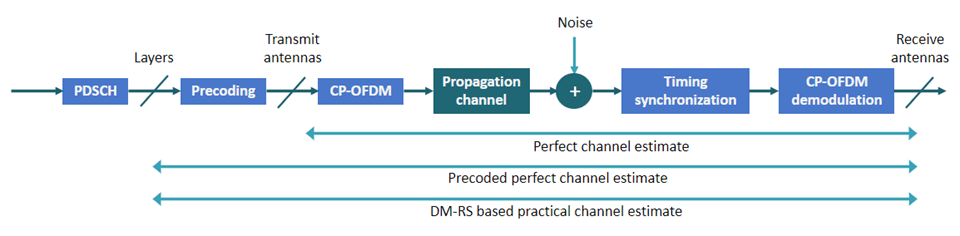Perform perfect or practical channel estimation.

``` if perfectEstimation % Perform perfect channel estimation between transmit and receive % antennas. estChGridAnts = nrPerfectChannelEstimate(carrier,pathGains,pathFilters,offset,sampleTimes); % Get perfect noise estimate (from noise realization) noiseGrid = nrOFDMDemodulate(carrier,noise(1+offset:end ,:)); noiseEst = var(noiseGrid(:)); % Get precoding matrix for next slot newPrecodingWeight = getPrecodingMatrix(pdsch.PRBSet,pdsch.NumLayers,estChGridAnts); % Apply precoding to estChGridAnts. The resulting estimate is for % the channel estimate between layers and receive antennas. estChGridLayers = precodeChannelEstimate(estChGridAnts,precodingWeights.'); else % Perform practical channel estimation between layers and receive % antennas. [estChGridLayers,noiseEst] = nrChannelEstimate(carrier,rxGrid,dmrsIndices,dmrsSymbols,'CDMLengths',pdsch.DMRS.CDMLengths); % Remove precoding from estChannelGrid before precoding % matrix calculation estChGridAnts = precodeChannelEstimate(estChGridLayers,conj(precodingWeights)); % Get precoding matrix for next slot newPrecodingWeight = getPrecodingMatrix(pdsch.PRBSet,pdsch.NumLayers,estChGridAnts); end```

Plot the channel estimate between the first layer and the first receive antenna.

` mesh(abs(estChGridLayers(:,:,1,1)));`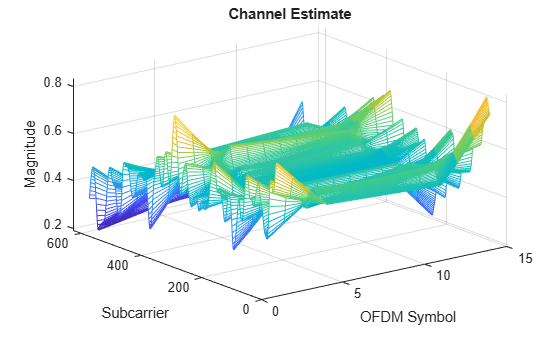``` title('Channel Estimate'); xlabel('OFDM Symbol'); ylabel("Subcarrier"); zlabel("Magnitude");```

At this point, you can use the channel estimate to obtain the precoding matrix for transmission in the next slot. Because this example assumes channel knowledge at the transmitter, you do not need to calculate the precoding matrix at the receiver end. For an example of how to calculate the precoding matrix for data transmission based on a channel estimate at the receiver, see NR PDSCH Throughput.

#### Equalization

The equalizer uses the channel estimate to compensate for the distortion introduced by the channel.

Extract the PDSCH symbols from the received grid and associated channel estimates. The `csi` output has channel state information (CSI) for each of the equalized PDSCH symbols. The CSI is a measure of the channel conditions for each PDSCH symbol. Use the CSI to weight the decoded soft bits after PDSCH decoding, effectively increasing the importance of symbols experiencing better channel conditions.

``` [pdschRx,pdschHest] = nrExtractResources(pdschIndices,rxGrid,estChGridLayers); [pdschEq,csi] = nrEqualizeMMSE(pdschRx,pdschHest,noiseEst);```

Plot the constellation of the equalized symbols. The plot includes the constellation diagrams for all layers.

``` constPlot.ChannelNames = "Layer "+(pdsch.NumLayers:-1:1); constPlot.ShowLegend = true; % Constellation for the first layer has a higher SNR than that for the % last layer. Flip the layers so that the constellations do not mask % each other. constPlot(fliplr(pdschEq));```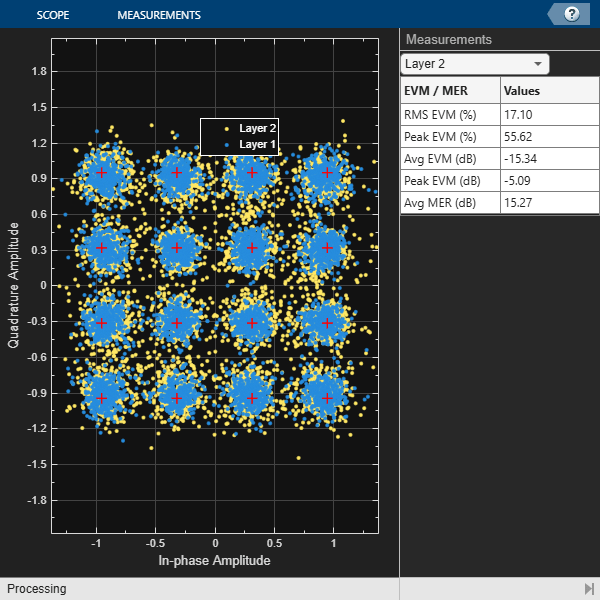#### PDSCH Decoding

Decode the equalized PDSCH symbols and obtain the soft bit codewords.

` [dlschLLRs,rxSymbols] = nrPDSCHDecode(carrier,pdsch,pdschEq,noiseEst);`

Scale the soft bits or log-likelihood ratios (LLRs) by the CSI. This scaling applies a larger weight to the symbols in the REs with better channel conditions.

``` % Scale LLRs by CSI csi = nrLayerDemap(csi); % CSI layer demapping for cwIdx = 1:pdsch.NumCodewords Qm = length(dlschLLRs{cwIdx})/length(rxSymbols{cwIdx}); % Bits per symbol csi{cwIdx} = repmat(csi{cwIdx}.',Qm,1); % Expand by each bit per symbol dlschLLRs{cwIdx} = dlschLLRs{cwIdx} .* csi{cwIdx}(:); % Scale end```

#### DL-SCH Decoding

Decode the LLRs and check for errors.

``` decodeDLSCH.TransportBlockLength = trBlkSizes; [decbits,blkerr] = decodeDLSCH(dlschLLRs,pdsch.Modulation,pdsch.NumLayers, ... harqEntity.RedundancyVersion,harqEntity.HARQProcessID);```

#### HARQ Process Update

Update the current HARQ process with the resulting block error status, and then advance to the next process. This step updates the information related to the active HARQ process in the HARQ entity.

` statusReport = updateAndAdvance(harqEntity,blkerr,trBlkSizes,pdschInfo.G); `

Summarize HARQ and decoding information for the present slot.

` disp("Slot "+(nSlot)+". "+statusReport);`
```Slot 0. HARQ Proc 0: CW0: Initial transmission passed (RV=0,CR=0.482212). Slot 1. HARQ Proc 1: CW0: Initial transmission passed (RV=0,CR=0.482212). Slot 2. HARQ Proc 2: CW0: Initial transmission passed (RV=0,CR=0.482212). Slot 3. HARQ Proc 3: CW0: Initial transmission passed (RV=0,CR=0.482212). Slot 4. HARQ Proc 4: CW0: Initial transmission passed (RV=0,CR=0.482212). Slot 5. HARQ Proc 5: CW0: Initial transmission passed (RV=0,CR=0.482212). Slot 6. HARQ Proc 6: CW0: Initial transmission passed (RV=0,CR=0.482212). Slot 7. HARQ Proc 7: CW0: Initial transmission passed (RV=0,CR=0.482212). Slot 8. HARQ Proc 8: CW0: Initial transmission passed (RV=0,CR=0.482212). Slot 9. HARQ Proc 9: CW0: Initial transmission passed (RV=0,CR=0.482212). Slot 10. HARQ Proc 10: CW0: Initial transmission passed (RV=0,CR=0.482212). Slot 11. HARQ Proc 11: CW0: Initial transmission passed (RV=0,CR=0.482212). Slot 12. HARQ Proc 12: CW0: Initial transmission passed (RV=0,CR=0.482212). Slot 13. HARQ Proc 13: CW0: Initial transmission passed (RV=0,CR=0.482212). Slot 14. HARQ Proc 14: CW0: Initial transmission passed (RV=0,CR=0.482212). Slot 15. HARQ Proc 15: CW0: Initial transmission passed (RV=0,CR=0.482212). Slot 16. HARQ Proc 0: CW0: Initial transmission passed (RV=0,CR=0.482212). Slot 17. HARQ Proc 1: CW0: Initial transmission passed (RV=0,CR=0.482212). Slot 18. HARQ Proc 2: CW0: Initial transmission passed (RV=0,CR=0.482212). ```
```Slot 19. HARQ Proc 3: CW0: Initial transmission passed (RV=0,CR=0.482212). ```
`end % for nSlot = 0:totalNoSlots`

### Local Functions

```function noise = generateAWGN(SNRdB,nRxAnts,Nfft,sizeRxWaveform) % Generate AWGN for a given value of SNR in dB (SNRDB), which is the % receiver SNR per RE and antenna, assuming the channel does % not affect the power of the signal. NRXANTS is the number of receive % antennas. NFFT is the FFT size used in OFDM demodulation. SIZERXWAVEFORM % is the size of the receive waveform used to calculate the size of the % noise matrix. % Normalize noise power by the IFFT size used in OFDM modulation, as % the OFDM modulator applies this normalization to the transmitted % waveform. Also normalize by the number of receive antennas, as the % channel model applies this normalization to the received waveform by % default. The SNR is defined per RE for each receive antenna (TS % 38.101-4). SNR = 10^(SNRdB/10); % Calculate linear noise gain N0 = 1/sqrt(2.0*nRxAnts*double(Nfft)*SNR); noise = N0*complex(randn(sizeRxWaveform),randn(sizeRxWaveform)); end function wtx = getPrecodingMatrix(PRBSet,NLayers,hestGrid) % Calculate precoding matrix given an allocation and a channel estimate % Allocated subcarrier indices allocSc = (1:12)' + 12*PRBSet(:).'; allocSc = allocSc(:); % Average channel estimate [~,~,R,P] = size(hestGrid); estAllocGrid = hestGrid(allocSc,:,:,:); Hest = permute(mean(reshape(estAllocGrid,[],R,P)),[2 3 1]); % SVD decomposition [~,~,V] = svd(Hest); wtx = V(:,1:NLayers).'; wtx = wtx/sqrt(NLayers); % Normalize by NLayers end function estChannelGrid = getInitialChannelEstimate(channel,carrier) % Obtain an initial channel estimate for calculating the precoding matrix. % This function assumes a perfect channel estimate % Clone of the channel chClone = channel.clone(); chClone.release(); % No filtering needed to get channel path gains chClone.ChannelFiltering = false; % Get channel path gains [pathGains,sampleTimes] = chClone(); % Perfect timing synchronization pathFilters = getPathFilters(chClone); offset = nrPerfectTimingEstimate(pathGains,pathFilters); % Perfect channel estimate estChannelGrid = nrPerfectChannelEstimate(carrier,pathGains,pathFilters,offset,sampleTimes); end function refPoints = getConstellationRefPoints(mod) % Calculate the reference constellation points for a given modulation % scheme. switch mod case "QPSK" nPts = 4; case "16QAM" nPts = 16; case "64QAM" nPts = 64; case "256QAM" nPts = 256; end binaryValues = int2bit(0:nPts-1,log2(nPts)); refPoints = nrSymbolModulate(binaryValues(:),mod); end function estChannelGrid = precodeChannelEstimate(estChannelGrid,W) % Apply precoding matrix W to the last dimension of the channel estimate. % Linearize 4-D matrix and reshape after multiplication K = size(estChannelGrid,1); L = size(estChannelGrid,2); R = size(estChannelGrid,3); estChannelGrid = reshape(estChannelGrid,K*L*R,[]); estChannelGrid = estChannelGrid*W; estChannelGrid = reshape(estChannelGrid,K,L,R,[]); end```